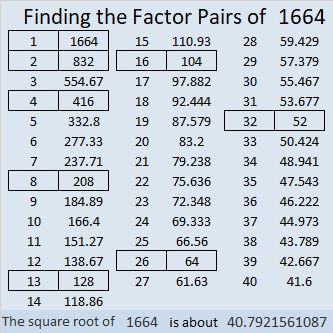# 1664 and Level 2

Contents

### Today’s Puzzle:

Write the numbers 1 to 12 in both the first column and the top row so that those numbers and the given clues function like a multiplication table.### Factor Cake for 1664:

We can make a factor cake for 1664 by doing some successive divisions. Divide 1664 by 2, divide that answer by 2, and so forth until you make a factor cake that looks like this:### Factors of 1664:

• 1664 is a composite number.
• Prime factorization: 1664 = 2 × 2 × 2 × 2 × 2 × 2 × 2 × 13, which can be written 1664 = 2⁷ × 13.
• 1664 has at least one exponent greater than 1 in its prime factorization so √1664 can be simplified. Taking the factor pair from the factor pair table below with the largest square number factor, we get √1664 = (√64)(√26) = 8√26.
• The exponents in the prime factorization are 7 and 1. Adding one to each exponent and multiplying we get (7 + 1)(1 + 1) = 8 × 2 = 16. Therefore 1664 has exactly 16 factors.
• The factors of 1664 are outlined with their factor pair partners in the graphic below.### More About the Number 1664:

1664 is the sum of two squares:
40² + 8² = 1664.
That happened because it has a prime factor that leaves a remainder of 1 when divided by 4 AND all of its other prime factors are powers of 2 or perfect squares:

But that’s not all that cool about 1664. What patterns do you notice below?
2(24² + 16²) = 1664,
4(20² + 4²) = 1664,
8(12² + 8²) = 1664,
16(10² + 2²) = 1664,
32(6² + 4²) = 1664,
64(5² + 1²) = 1664, and
128(3² + 2²) = 1664.

1664 is the hypotenuse of a Pythagorean triple:
640-1536-1664, calculated from 2(40)(8), 40² – 8², 40² + 8².
That triple is also (5-12-13) times 128.

This site uses Akismet to reduce spam. Learn how your comment data is processed.